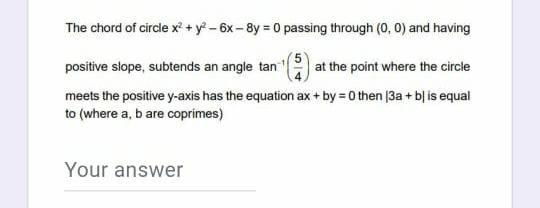Question:

# The chord of circle x2 + y - 6x-8y = 0 passing through (0,0) and having positive slope, subtends an angle tan at the point whereThe chord of circle x2 + y - 6x-8y = 0 passing through (0,0) and having positive slope, subtends an angle tan at the point where the circle meets the positive y-axis has the equation ax + by =0 then 3a + b} is equal to (where a, b are coprimes) Your answer